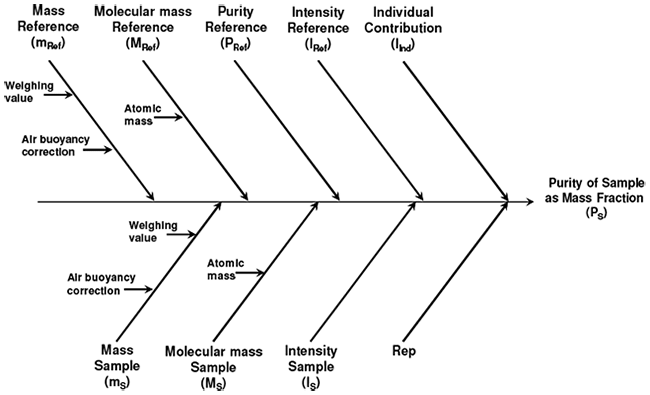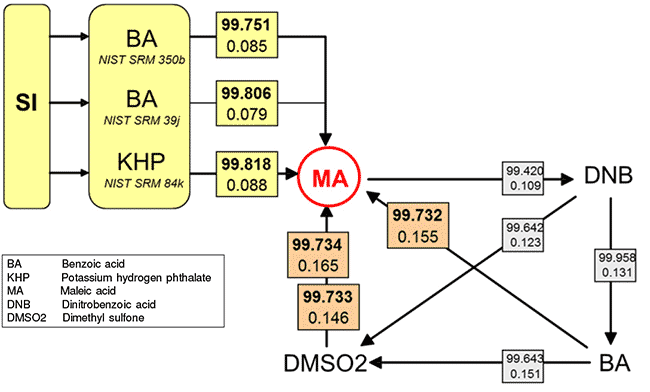HomeCalibration, Qualification & ValidationHigh-Performance Quantitative H-1 NMR

# High-Performance Quantitative H-1 NMR

Markus Obkircher , Christine Hellriegel , Alex Rueck , Juerg Wuethrich , Michael Weber , Jiewen Ying

## Introduction

Quantification of organic substances is usually performed using chromatographic techniques such as HPLC or GC. Over the last years quantitative NMR (qNMR) has evolved not only in pharmaceutical industry but also in many other fields and gains increasing interest as alternative quantification method . The most outstanding attribute of qNMR is that it is a relative primary method because the signal intensity is in direct proportionality with the number of protons contributing to the resonance . Hereby the structures of the chemical substances are fully irrelevant. Since signal intensities of analyte and reference substance can be compared directly, a direct traceability to internationally accepted reference standards (e.g. NIST SRM), and therefore to SI units, can be achieved , which is usually not possible with chromatographic techniques.

The signal ratio of two different protons can be measured with tremendous precision resulting in high trueness and low uncertainties, which enables the generation of certified reference material (CRM) for use as qNMR standards for proton NMR. Buoyancy correction was implemented into the content calculation and the final certificate contains a proper uncertainty calculation , comprehensive documentation and storage information based on stability studies . All experiments are performed under ISO/IEC 17025 and ISO Guide 34 accreditation. Figure 1.Figure 1. Cause-Effect Diagram of the Uncertainty Contributions for the qNMR Measurement

## Validation Experiments: Different traceability chains for Maleic acid

In order to show the robustness of qNMR results, we set up the following experiment (Figure 2). Maleic acid (MA) was chosen as a (secondary) calibrator and its purity (expressed as mass fraction) was quantified by 1H-qNMR with respect to three different (primary) reference materials: NIST SRM 350b Benzoic acid (NIST-BA1), NIST SRM 39j Benzoic acid (NIST-BA2), and NIST SRM 84k Potassium hydrogen phthalate (NIST-KHP).

Exp. A: MA was quantified using NIST-BA1 as primary calibrator

Exp. B: MA was quantified using NIST-BA2 as primary calibrator

Exp. C: MA was quantified using NIST-KHP as primary calibrator

Three independent, different values for the mass fraction of MA were obtained, whereby “value” always describes the assigned purity expressed as mass fraction including its expanded uncertainty at the 95% confidence level (k = 2). Based on these values, the MA was then taken as the calibrator for the following measurement series:

Exp. D: DNB was quantified using MA as calibrator. Then DNB was taken as the calibrator for BA. Finally, BA was taken as the calibrator for MA.

Exp. E: DNB was quantified using MA as calibrator. Then DNB was taken as the calibrator for DMSO2. Finally, DMSO2 was taken as the calibrator for MA.

Exp. F: DNB was quantified using MA as calibrator. Then DNB was taken as the calibrator for BA. Then BA was taken as the calibrator for DMSO2. Finally, DMSO2 was taken as the calibrator for MA.Figure 2.Validation of qNMR Measurement Results for the Certification of Maleic Acid Purity Through Six Different Traceability Chains. Upper values refer to the assigned purity expressed as mass fraction in %, lower values refer to the corresponding expanded absolute uncertainty of the mass fraction value in % (k=2). The six different values assigning a purity value for MA are given in bold face. The comparability of these six measurement results is further illustrated in Figure 3.

Validation of qNMR measurement results with focus on accuracy and robustness. Maleic acid was quantified in 6 different ways, directly and indirectly (Figure 3). Upper values refer to the assigned purity expressed as mass fraction in %, lower values refer to the corresponding expanded absolute uncertainty of the mass fraction value in % (k=2).

• 3 results came from a direct measurement against a primary calibrator (Exp. A,B,C)
• 2 results were generated by a 3-step comparison measurement (Exp. D+E)
• 1 result was observed from a 4-step comparison measurement (Exp. F)
• all 6 results show full consistency in terms of overlapping of the uncertainty budgets with all the other mean values
• the relative standard deviation of the 6 mean values from Exp. A to F is 0.04 % and all the 6 measurement results are covered within a range of +/- 0.05 %
• the direct measurements (Exp. A to C) show the lowest uncertainties (all below 0.09 % relative) and due to uncertainty propagation, the uncertainty values are higher for Exp. D to F
• Exp. F comprises 4 comparison measurements and therefore shows the highest uncertainty. Nevertheless, Exp. F shows the enormous potential of the 1H-qNMR method: the content of MA was confirmed within an expanded relative standard uncertainty of < 0.2 % although this value was generated over a series of 4 comparison measurements using 3 intermediate referencesFigure 3.Comparison of Purity Results of Maleic Acid

## Influence of Signal Intensity and Pattern

Some molecules offer more than 1 signal for purity determination and also different signal patterns. Using maleic acid as internal calibrator, the purity of Ethyl 4-(dimethylamino)benzoate (EDAB) was assigned evaluating 4 different protons. Two experiments were realized with different optimized ratios of signal intensities between EDAB and calibrator (Figure 4). The optimal ratio of 1:1 signal intensity for each experiment is indicated by a black dot.

Exp. 1: The mass ratio of sample and calibrator was optimized to obtain a 1:1 peak ratio of MA and the 6 methyl protons of the amino group of EDAB
Exp. 2: The mass ratio of sample and calibrator was optimized for a 1:1 peak ratio of MA and the ethyl group of EDAB (2 isochronic nuclei)

Results:

• almost identical values of the 3 results shown with black dots
• all 8 values show good consistency in the range of +/- 0.07 % relative standard deviation of the mean values
• the signal pattern (singlet vs. multiplet) seems not to be of highest relevance in terms of accuracy (see 3 black dots)Figure 4. EDAB Purity Assignments using MA Calibrator

## Summary

The results show that 1H-qNMR, combined with metrological weighing, can be optimized to obtain results to certify the purity of organic reference materials (expressed as mass fraction) with less than 0.1 % relative expanded uncertainty for a 95 % confidence interval (k=2). The validity, accuracy and robustness of the technique was proven by a series of experiments. The purity of maleic acid was determined by 6 different 1H-qNMR measurement series and all results showed full consistency. All the 6 mean values are covered within the range of +/- 0.05 %. 1H-qNMR measurement results are directly traceable to a variety of internationally accepted primary reference materials, and therefore traceability to SI units is obtained.

Materials